#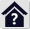Flywheels

## Flywheels

The program is intended for a design of a flywheel, its analysis, and determination of dimensions. It also allows to solve a crank mechanism and to determine parameters of asynchronous motors.

The program solves:

1. Design of a moment of inertia for a selected machine
2. Design of flywheel dimensions based on a required moment of inertia
3. Calculation of a moment of inertia based on dimensions of a flywheel
4. Calculation of a moment of inertia of masses with different revolutions (reduced moment of inertia)
5. Calculation of a stress in rotating rings
6. Calculation of gyroscopic moment
7. Calculation of a moment of inertia from a load diagram and a required run unevenness
8. Calculation of coefficient of fluctuation of speed
9. Design of a flywheel in connection with a motor
10. Selection and calculation of parameters of approximately 1200 asynchronous electric motors
11. Crank mechanism solution

The calculations use data, procedures, algorithms and information from literature, standards, and company catalogues.
 Strojně technická příručka (Svatopluk Černoch)
 Shigley’s Mechanical Engineering Design
 Strojírenská příručka (1992)

Machinery’s Handbook (26th Edition)

Standards: EN 60034-30-1 :20 14
Rotating electrical machines — Part 30-1: Efficiency classes of line operated AC motors (IE code)
Machines Olectriques tournantes — Partie 30-1: Classes de rendement pour les moteurs a courant alternatif alimentés par le réseau (Code IE)
Drehende elektrische Maschinen — Teil 30-1: Wirkungrad-Klassifizierung von netzgespeisten Drehstrommotoren (IE-Code)

Company cataloques:Siemens, ABB Automation Products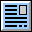User interface.

Purchase, Price list.

## Control, structure and syntax of calculations.

Information on the syntax and control of the calculation can be found in the document "Control, structure and syntax of calculations".

## Information on the project.

Information on the purpose, use and control of the paragraph "Information on the project" can be found in the document  "Information on the project".

## Theory.

### Design and Calculation of Moment of Inertia and Flywheel Dimensions

The following formulas are used in the calculations.

#### Approximate Design of a Moment of Inertia of a Flywheel

I = C * Pw / (n³ * δ)

C ... Güldner's constant, which determines the type of machine
Pw ... Machine power
n ... Apeed [/s]
δ ... Coefficient of fluctuation of speed recommended for the machine type

#### Coefficient of Fluctuation of Speed

δ = (ωmax - ωmin) / ωm

ωmax ... Maximum angular speed
ωmin ... Minimum angular speed
ωm = (ωmax - ωmin) / 2 ... Mean angular velocity

#### Moment of Inertia of the Rotating Disc

I = 0.5 * Ro * Pi * b * (R⁴ - r⁴)

Ro ... Density
b ..... Disc width

#### Reduced Moment of Inertia

If there are masses in the system rotating at different speeds (transmission ratio), it is necessary to convert their moment of inertia to the moment of inertia of the flywheel.

Ired = Σ I(i) * (n / n(i))²
I(i) ... The moment of inertia of a mass rotating at a different speed than the flywheel
n ...... Flywheel speed
n(i) ... The rotational moment of a mass rotating at a different speed than the flywheel
The reduced moments of inertia can then be added directly to find the total moment of inertia of the system.

#### Tangential stress

σt = Ro * ω² * ((3 + ny) /8 ) * (r² + R² + r² * R² / rx² - (1 + 3 * ny) / (3 + ny) * rx²)

Ro ... Density
ω ... Angular velocity
ny ... Poisson's constant
rx .... The radius at which the stress is calculated

#### Spin of the flywheel

Time
t = (0.1047197533 * (n2 - n1) * I) / T

Energy
E = 0.005483 * I * (n2² - n1²)

T ... Torque
I .... Moment of inertia
n1,n2 ... Speed

#### Gyroscopic Moment

Tg = Pi * n * I * ωv / 30 * sin(β)

I .... Moment of inertia of the flywheel
n ... Flywheel speed [/min]
β ... Angle of the flywheel rotation axis
ωv ... Angular speed of rotation of the flywheel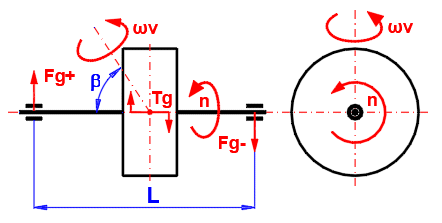### Analysis (calculation) of Flywheel

In motors and machines where rotary motion is converted to rectilinear and in machines where there is no constant load, torque fluctuation occurs. There is no constant balance between the acting moments (driving, driven, inertial forces, passive resistances). This results in a change in angular velocity during the work cycle. To reduce this inequality, a flywheel is used as an energy accumulator, which accumulates the energy in the event of an excess of torque, and releases it in the event of a lack of it.

A large number of machines have a torque curve that cannot be simply described analytically. Therefore, numerical integration methods are used for the solution. The torque sequence on the left side is defined by the torque table in dependence on the rotation of the flywheel. The moment on the right side can be:
A. Constant
B. Dependent on the speed of the flywheel (electric motor, generator)
C. Or it can be defined by a custom table or function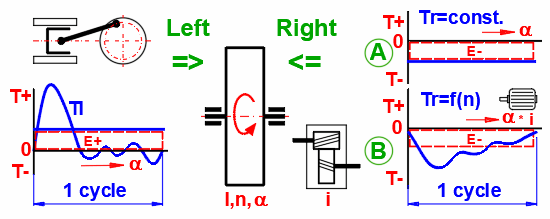#### Basic equation of motion: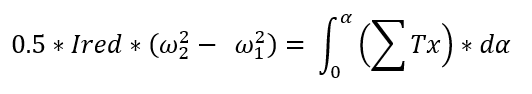Ired … Reduced moment of inertia of all rotating masses
ω1, ω2 … Angular velocity at the beginning and end of the work cycle
a … Angle of rotation of the flywheel during the working cycle
da … Elementary rotation of the flywheel
ΣTx ... The sum of all acting moments (Driving, driven, inertial forces, passive resistances)

The goal is to solve the course of the acting moment on the right side so that it meets the conditions (e.g. the characteristics of an asynchronous electric motor) and at the same time the sum of the energies of the left and right sides is zero (ω1 = ω2).

An example can be the dependence of the torque of a single-cylinder four-stroke internal combustion engine on the angle of rotation of the crank (see picture).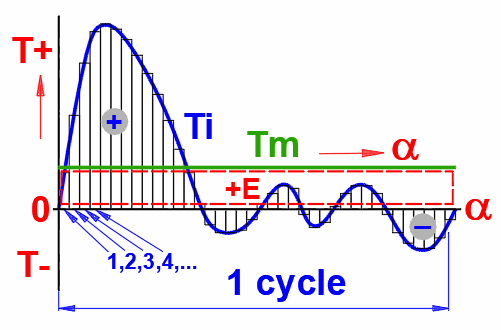The calculation takes place in the following steps:

#### 1) Integration and Energy Calculation (Division of the Ti course into n parts)

Tmid(j) = (Ti(j) + Ti(j+1)) / 2 ... <j = 1 ... n>

dE(j) = Tmid(j) * da ... <j = 1 ... n> ... for each segment 1,2,3,4.....

Where:
da = a / n
dEsum = ΣdE(j) ... <j = 1 ... n>

Tm = dEsum / a

#### 3) Angular Velocity at Each Point

ω(j+1) = (ω(j) + 2 * dE'(j) / I)^0.5

where:
dE'(j) = (Tmid(j) - Tm) * da
I ... The moment of inertia of the flywheel and the attached rotating masses

#### 4) Finding the ωmin and ωmax Angular Velocities from the Field ω(j) ... <j = 1 ... n+1>

ωmin = MIN (ω(j)) ... <j = 1 ... n+1>
ωmax = MAX (ω(j)) ... <j = 1 ... n+1>

#### 5) Calculation of the Mean Angular Velocity

ωm = (ωmin + ωmax) / 2

#### 6) Calculation of the Coefficient of Fluctuation of Speed

δ = (ωmax - ωmin) / ωm

Alternatively, calculate the moment of inertia from the coefficient of fluctuation of speed.
I = dEsum' / (δ * ωm^2)

where:
dEsum' = ΣdE'(j) ... <j = 1 ... n>If, for example, an asynchronous motor (generator) is used instead of a constant value Tm, the torque of which is dependent on the speed, the torque value, which corresponds to the revolutions of the flywheel for each step, is gradually used. The entire model is recalculated with each change.

In dependence on the input data (motor power, moment of inertia, degree of non-uniformity, etc.) the solution converges to equilibrium usually in the matter of several tens of iterations.

Analysis allows you to solve/examine:
- Accurate calculation of moment of inertia for the required degree of run unevenness
- Calculation of run unevenness for the specified moment of inertia
- Calculation of the course of revolutions, moments, energies, and powers
- Suitability check of the selected motor/generator (speed and power)

An example of analysis of an asynchronous generator coupled to a single-cylinder gasoline engine for various non-uniformities of the delta run: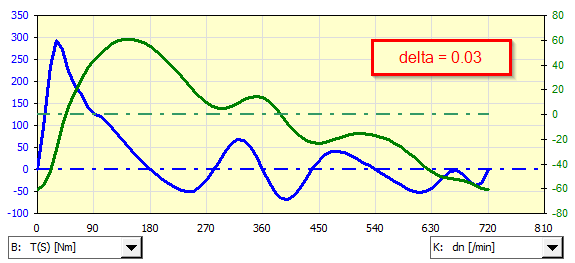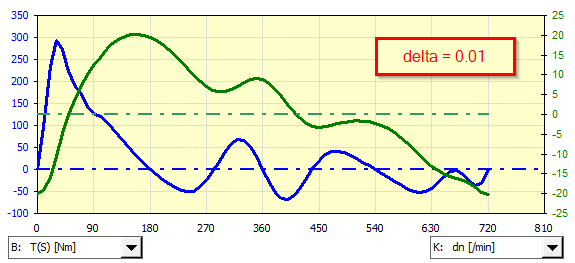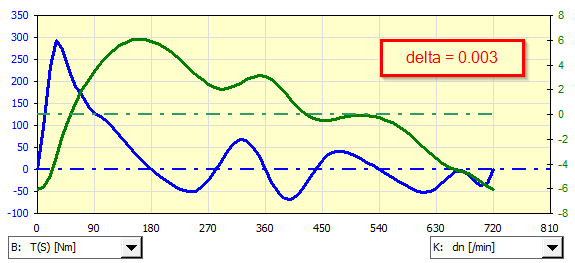If it is necessary to use a non-predefined asynchronous motor (generator), its characteristic can be defined manually and used during iteration.

### Motor Torque Curves

In the field of machine and equipment drives, an asynchronous motor (induction motor) is most often used. For this motor, the torque changes with the revolutions (linearly in the working range). A table of parameters for any motor was generated by comparing numerous manufacturers’ catalogs.

In this chapter, you will find the parameters of the selected motor (including estimation of weight, efficiency and moment of inertia), power and moment for other than nominal speeds.

#### Basic Formulas for Induction Motors

Synchronous speed
ns = 2 * f * 60 / p
f ... Frequency of the power grid (60 Hz USA, Canada..., 50 Hz Europe)
p ... Number of motor poles

Rated speed (Asynchronous speed)
nr = (1 - s / 100) * ns
s ... Motor slip [%]motor

Rated torque
Tr = Pr * 9550 / nr
Pr ... rated power

Pull-up torque
Tz = Tr * Tzcoeff
Tzcoeff ... Pull-up torque coefficient
For small motors (≤ 30 kW) the value is 2.5-3, for medium motors 2-2.5, for large motors 1-1.5.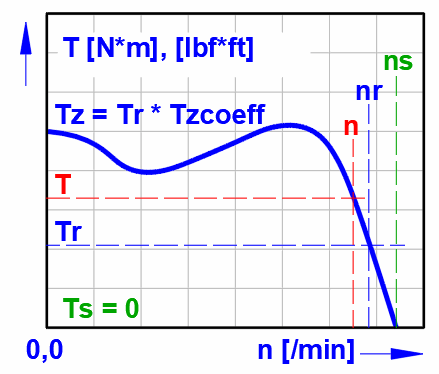### Crank Mechanism

A crank mechanism is often used to convert rectilinear to rotary motion (and vice versa), usually in combination with a flywheel. For the design of the flywheel, it is then necessary to know the course of the moment. To calculate the course of the moment, it is necessary to know the course of the loading force (Fp), the dimensions (L,R), the revolutions (n) and the weight of the individual parts (mp, m).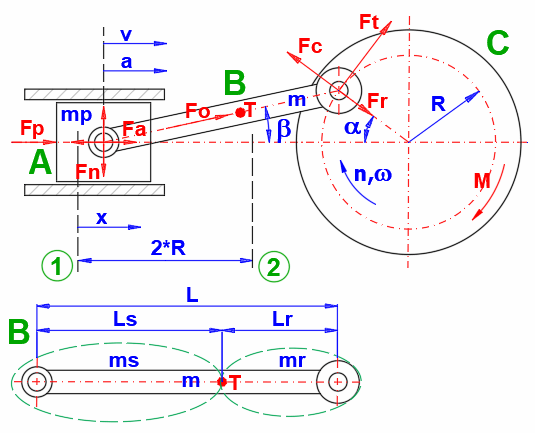The following formulas are used in the calculation to determine the course of the moment during crankshaft rotation. The meaning of the individual variables is shown in the figure.

β = asin(R / L * sin(α))
λ = R / L
x = R * (1 - cos(α) + λ / 2 * sin(α)^2) ... Distance from the top pivot (1)
v = R * ω * (sin(α) + λ * sin(α) * cos(α))
a = R * ω^2 * (cos(α) + λ * cos(2 * α))
ω =2 * Pi * n / 60
ms = Lr / L * m
mr = Ls / L * m
Fa = (m + ms) * a ... Acceleration/Deceleration of straight moving parts
Fn = Fp * tan(β) + Fa * tan(β)
Ft = Fp * (sin(α) + λ * sin(2 * α) + Fa * (sin(α) + λ * sin(2 * α)
Fo = Fp / cos(β)
Fr = Fo * cos(α + β)
Fc = mr * R * ω^2 ... Centrifugal force of connecting rod on crank
M = R * Ft ... Moment from load force Fp and acceleration/deceleration force Fa

## Calculation procedure:

The calculation is intended for a quick flywheel design and its analysis.

Aproximate design, paragraph :
- Approximate design of a moment of inertia of a flywheel according to a type and parameters of the machine
- Design of flywheel dimensions based on a moment of inertia
- Voltage control, max. speed control, gyroscopic moment control

Detailed analysis, paragraph :
If you know the torque curve of the machine for which you want to design a flywheel, use the table to define the curve. You will quickly find out the necessary moment of inertia for the required run unevenness, or the run unevenness for the entered moment of inertia (Analysis A).

Use Analysis B, if you need to solve a pair of machine x electric motor (machine x generator).

## Calculation units, units conversion 

In this paragraph you set the units of calculation.

### 1.1 Calculation units

Select the desired system of calculation units in the list box. After switching over the units, all values will be changed immediately.

### 1.2 Units conversion

Type the value you want to convert into the input box on the left. Select source units and target units on the right.

## Design and Calculation of Moment of Inertia, Flywheel Dimensions and Gyroscopic Moment 

- Approximate design of a moment of inertia for a selected type of machine
- Design of flywheel dimensions based on moment of inertia
- Calculation of a moment of inertia from dimensions of a flywheel
- Calculation of energy of a rotating flywheel and calculation of the maximum voltage
- Recalculation of moments of inertia of masses rotating at different speeds
- Calculation of a time necessary to to spin the flywheel
- Calculation of gyroscopic moment and forces

### 2.1 Approximate Design of Flywheel Moment of Inertia

It is possible to estimate the necessary moment of inertia for a flywheel based on the type of machine, the power, and the speed of the flywheel.
Select the type of machine (and thus the constant C that characterizes it), enter the nominal power, the speed of the flywheel, and the required degree of unevenness.

### 2.6 Coefficient of fluctuation of speed

The following values are recommended for different areas of machinery.

Type of machine / coefficient of fluctuation of speed (δ)
Crushing machines ..... 0.200
Electrical machines ..... 0.003
Electrical machines (direct drive) ..... 0.002
Engines with belt transmission ..... 0.030
Gear wheel transmission ..... 0.020
Hammering machines ..... 0.200
Pumping machines ..... 0.030 to 0.05
Machine tools ..... 0.030
Paper making ..... 0.015
Textile and weaving machines ..... 0.010 - 0.025
Punching. shearing and power presses ..... 0.10 - 0.15
Spinning machinery ..... 0.10 - 0.020
Rolling mills and mining machines ..... 0.025
DC generators ..... 0.006 - 0.013
AC generators ..... 0.003 - 0.015
Vehicle internal combustion engines ..... 0.003 - 0.006

### 2.8 Preliminary Design of Flywheel Dimensions

Based on the required moment of inertia, you can design the dimensions of the flywheel ring.
Enter the required moment of inertia, the density of the material used, the ratio of the width and height of the flywheel rim, and the required inner diameter.

### 2.9 Required Moment of Inertia

The moment of inertia from the reference design is used. If you want to enter a custom value, uncheck the checkbox on the right.

### 2.10 Density of flywheel material

Steel 7800 [kg/m³]; 487 [lb/ft³]
Cast iron 7200 [kg/m³]; 450 [lb/ft³]
Bronze 8800 [kg/m³]; 550 [lb/ft³]
Aluminum 2700 [kg/m³]; 168 [lb/ft³]
Hardwood 680 [kg/m³]; 42 [lb/ft³]

### 2.18 Maximum Speed, Maximum Stress

The greatest stress in a rotating ring is the tangential stress on its inner side.
Enter the maximum allowable stress and Poisson's constant of the material.
Enter RPM. The green field shows the maximum speed value for the entered maximum permissible voltage.
Pressing the "==>" button transferrs the calculated design values into the selected row of the table.

### 2.19 Maximum allowable stress

Cast iron ... < 8-10 MPa (1.16 - 1.45 kpsi)
Casting steel ... < 100 MPa (14.5 kpsi)

### 2.20 Poisson's constant

Steel ... 0.27-0.30
Cast iron ... 0.21-0.26
Aluminium alloys ... 0.33
Copper ... 0.33
Magnesium ... 0.35
Titanium ... 0.34

### 2.21 Flywheel Speed

The revolutions value from line [2.5] is used as the default. To enter your own values, uncheck the checkbox to the right.

### 2.23 Tangential Stress on Inner Diameter

The calculated stress should be less than the maximum allowed [2.19].

### 2.25 Dimensions and Calculation of Flywheel (Inertial Masses)

You can define up to 12 different rings in the table and thus assemble a more complex flywheel shape, or recalculate masses with different rotation speeds.
The "Clear" button clears the dimensional values in the entire table, except for the first row.
The "V" button transfers the value from the first line to the others.
The "min/max" button fills in the outer/inner diameter of the ring to calculate the stress on the outer/inner diameter.

###### Tip: If other rotating masses (gears, pulleys, etc.) are connected to the flywheel by means of a gear, you can add them by defining them in the table and assigning them the corresponding revolutions. The total reduced moment of inertia will be converted by the gear ratio to the first line and is in the "Ired" column.

Column labels
Number: Multiplies calculated values (multiple identical rotating masses)
a: Displacement of the ring (cylinder) in the graph in the X axis
D, d, b: Ring (cylinder) dimensions
Ro: Density
ny: Poisson's constant
n: RPM
ω: Angular velocity
m: Mass
I: Moment of inertia
Ired: Reduced moment of inertia (recalculated via revolutions to the first line)
Ek: Kinetic energy of the rotating disc
dx: The ratio for which the tangential stress will be calculated
σt: Tangential stress

### 2.26 Turning the Flywheel

Calculation of the energy and time required to spin the flywheel with the specified moment of inertia to the required speed.
The values are preset from the table above. To enter your own values uncheck the checkbox to the right.

### 2.33 Gyroscopic Moment

When the flywheel rotates around an axis other than the axis of rotation, a gyroscopic moment is generated. For larger moments of inertia and higher speeds, it can be significant and must be taken into account in the calculations.
The preset values are from the table above, after unchecking the checkbox you can enter your own values.

### 2.41 Graph

Objects from table [2.25] are plotted in the graph.
The "a" coordinate in the table defines the displacement in the X axis.

## Analysis (calculation) of Flywheel 

In motors and machines where rotary motion is converted to rectilinear and in machines where there is no constant load, torque fluctuation occurs. There is no constant balance between the acting moments (driving, driven, inertial forces, passive resistances). This results in a change in angular velocity during the work cycle. To reduce this inequality, a flywheel is used as an energy accumulator, which accumulates the energy in the event of an excess of torque, and releases it in the event of a lack of it.

A large number of machines have a torque curve that cannot be simply described analytically. Therefore, numerical integration methods are used for the solution. The torque sequence on the left side is defined by the torque table in dependence on the rotation of the flywheel. The moment on the right side can be:
A. Constant
B. Dependent on the speed of the flywheel (electric motor, generator)
C. Or it can be defined by a custom table or function

!!! Proceed as follows during the analysis !!!
1. Fill in the table [3.45] with the course of the moment of the analyzed machine
2. Enter flywheel parameters [3.1]
3. Run analysis A or B or C
4. Review the results of the analysis on lines [3.28 - 3.42], in the table [3.45] and in the graphs

### 3.1 Required Flywheel Parameters

You can either enter the moment of inertia and the result of the analysis will be the degree of running unevenness, or by unchecking the checkbox on the right, you can enter the degree of running unevenness, and the result will be the corresponding moment of inertia.

### 3.3 Coefficient of fluctuation of speed

The following values are recommended for different areas of machinery.

Type of machine / coefficient of fluctuation of speed (δ)
Crushing machines ..... 0.200
Electrical machines ..... 0.003
Electrical machines (direct drive) ..... 0.002
Engines with belt transmission ..... 0.030
Gear wheel transmission ..... 0.020
Hammering machines ..... 0.200
Pumping machines ..... 0.030 to 0.05
Machine tools ..... 0.030
Paper making ..... 0.015
Textile and weaving machines ..... 0.010 - 0.025
Punching. shearing and power presses ..... 0.10 - 0.15
Spinning machinery ..... 0.10 - 0.020
Rolling mills and mining machines ..... 0.025
DC generators ..... 0.006 - 0.013
AC generators ..... 0.003 - 0.015
Vehicle internal combustion engines ..... 0.003 - 0.006

### 3.4 Required Mean Flywheel Speed

Enter the required flywheel speed.

### 3.7 A. Analysis - Constant Moment (Rright Side)

Basic analysis, applicable in all cases.

In this calculation, the increase (decrease) in energy is calculated from the moment curve on the left. From the calculated energy, a constant moment is determined, which must act on the right side so that the sum of the energies is zero.

On the basis of the acting moments (left + right side), the course of the angular velocity (revolutions) and thus the unevenness of the run for the specified moment of inertia of the flywheel is obtained. Or the moment of inertia of the flywheel for a given degree of unevenness is determined.

### 3.8 Power (Input)

Power + (power input -) device on the right, to generate the required constant torque (mean torque Tm).

### 3.10 B. Analysis - Electric Motor / Generator (Right Side)

Special case analysis where an asynchronous motor/generator (right side) is connected to the device (left side).

In addition to the condition of the sum of the energies of the left and right sides is equal to zero, it is also necessary to observe that the torque of the electric motor/generator is a function of the flywheel revolutions.

In this calculation, the motor torque is used repeatedly (see the number of iteration steps) according to the current flywheel revolutions, while the entire cycle is recalculated after each individual change. After each iteration step, the flywheel speed at the beginning of the cycle is adjusted accordingly. Most of the time, the whole model converges fast enough for smaller tens of iteration steps.

### 3.12 / 4.2 Type of Motor / Generator

Select a motor from the list.

Motors are listed in the form of “<Synchronous speed> / <number of poles> ... <frequency of the electrical system>"

### 3.13 Recommended Rated Power From / To

Recommended motor (generator) power interval estimated from the torque curve on the left.

### 3.14 / 4.3 Rated Power

Enter the motor power. Approximate motor parameters (nr, Tz, etc.) will be proposed based on the power.

Catalog values of motor manufacturers:

kW (50Hz):
0.06; 0.09; 0.12; 0.18; 0.25; 0.37; 0.55; 0.75; 1.1; 1.5; 2.2; 3; 3.7; 4; 5.5; 7.5; 9; 11; 15; 18.5; 22; 30; 37; 45; 55; 75; 90; 110; 132; 160; 200; 225; 250; 280; 315; 355; 400; 450; 500; 560; 630; 710; 800; 900; 1000

HP (60Hz):
0.25; 0.33; 0.5; 0.75; 1; 1.5; 2; 3; 4; 5; 5.5; 7.5; 10; 12; 15; 20; 25; 30; 40; 50; 60; 75; 100; 120; 150; 180; 210; 270; 300; 335; 375; 420; 475; 530; 600; 670; 750; 850; 950

### 3.15 / 4.4 Mode of Work

The motor can also function as an electric current generator (Exceeding synchronous speed and consumption of mechanical energy).

Select from the list to set the type of behavior.

### 3.16 / 4.5 Synchronous Speed

Set according to the selected motor type. At these speeds there is zero torque.

### 3.17 / 4.6 Rated Speed

The rated speed and starting torque coefficient are directly given for the specific motor. The green field contains an approximate value for the given motor type and power.
To enter your own values (nr, Tzcoeff), uncheck the checkbox on the right. It is thus possible to set the motor characteristics exactly according to the catalog of a specific manufacturer.

### 3.19 / 4.8 Pull-up Torque Coefficient

For small motors (≤ 30 kW) the value is 2.5-3, for medium 2-2.5, for large 1-1.5.

### 3.21 Moment of Inertia of the Motor / Ie * i

The first number indicates the approximate moment of inertia of the selected motor. The second number is multiplied by the square of the gear ratio "i" from the next line. It is thus possible to directly compare or subtract the value from the moment of inertia of the flywheel.

### 3.22 Gear Ratio Flywheel / Motor

The gear ratio is set automatically. It is estimated so that the required mean flywheel speed [3.4] approaches the calculated one.

To enter your own values, uncheck the checkbox on the right. This can be convenient if you need to set, for example, a gear ratio i=1 (direct flywheel drive).

### 3.25 Number of Iteration Steps / Sensitivity

Iteration
Set the number of iteration steps. After each step, the table and graph are refreshed, thus it is possible to follow the gradual changes.

###### Tip: Each iteration step takes up to a few seconds. For an initial idea of the model behavior, about 5-10 steps should be enough. After the end of the cycle, you can start another analysis, which will continue from the last values.

Sensitivity
The sensitivity determines the amount of change in the initial speed of the flywheel before each step.
The larger the value, the larger the change and the faster the convergence. On the other hand, too large a value can lead to oscillation or non-functional solution.

### 3.27 Analysis Results

The letter indicates for which analysis (A, B, C) the results are shown.

### 3.32 Sum of Energy+- / Deviation in Percent

The sum of the energies from the left side and from the right side of the flywheel. For steady operation (flywheel speeds at the beginning and end of the duty cycle are the same), the sum must be close to zero. The percentages express the deviation of the sum from the input energy (left side). A red value means that the deviation is greater than 1%.

### 3.33 Flywheel

Summary results of the flywheel calculation.

### 3.34 Mean Speed of Flywheel

The default setting of the average flywheel speed for the calculation and the angular velocity calculated from them.

Analysis A: The value from the required revolutions is set in the cell [3.4]
Analysis B, C: The corrected value from the previous step is moved to the cell after each iteration step (green cell).

### 3.43 Table of Moments Definition (left side)

Before starting the analysis, it is necessary to fill in the torque curve of the machine that we want to analyze (left side).

Define the flywheel load in the first two columns A, B of the table.

1) In column A, enter consecutively the angles for which you will define the moment acting on the flywheel (left side).
- Values must be in a continuous row without empty lines
- Angles must be entered in ascending order
- The difference between subsequent angles may not be regular. This can be used for example during a step change in torque (the difference between the subsequent angles is small)

2) Enter the torque acting on the flywheel in column B. A positive value speeds up the flywheel; a negative value slows the flywheel down.

### 3.44 Number of Valid Table Rows

It is necessary to enter the number of valid rows of the table (those that will be included into the analysis).
If you want to enter a value other than the suggested one (green cell), uncheck the checkbox.
The suggested value follows the first empty cell in column B.

### 3.45 Loads and Results Table

You have defined the moment load in column A,B (left side) of the table. The other columns are the result of the selected analysis.

α: The angle of rotation of the flywheel for which the moment is defined in column B. By pressing the "+Δ" button, the entire column is filled so that each subsequent value is increased by the difference between the second and the first line.

###### Note: The difference between the angles does not need to be regular, but must be ascending.

Tl: Torque curve of the machine you want to analyze (left side)

Ti=Tl+Tr: Course of the moment acting on the flywheel. Sum of the left and right sides.

Tr=Tm: The torque curve that balances the torque from column B
Analysis A: Constant, Tr = Tm
Analysis B: Iteration, Tr = Te * i
Analysis C: User Defined Moment

ne: Motor speed dependent on flywheel speed. ne = n * i

Te: Motor torque. It depends on the speed ne.

Pwe: Motor power.

+-dE: Energy that is supplied (+) / removed (-) to the flywheel between the current and the next angle of rotation (column A)

E: Current value of kinetic energy at the relevant angle of rotation of the flywhee.

n: RPM for the respective angle of rotation of the flywheel

dn: Difference between current and target revolutions

ω: Angular velocity at the respective roll angle

dω: Fluctuation of angular velocity. The difference between the current speed for the respective roll angle and the mean value

Pw: Flywheel power for a given angle of rotation

### 3.46 Graph 1 - Motor

Torque and power curve of the electric motor / generator
Axis: X - RPM, (red vertical = ns)
Axis: Y
Blue: Torque [Nm; lbf*ft] + work area
Green: Power [kWh; HP] + work area

### 3.47 Graph 2 - Torque, Speed

Moments and speed
Axis: X - Spin the flywheel
Axis: Y
Light black: Moment left side [Nm; lbf*ft]
Light red: Moment right side [Nm; lbf*ft]
Strong blue: Sum of moments [Nm; lbf*ft]
Strong green: flywheel speed [/min] (scale on the right)

### 3.48 Graph 3 - Selected Values

Select the curves you want to display in the puldowns below the graph.
Axis: X - Spin the flywheel

### 3.49 C. User Analysis

The three columns on the left in the table can be used for user definition of the moment (right side); for example in dependence on the speed of the flywheel.
Clicking the buttons "Tr=O(i)", "Tr=P(i)", or "Tr=Q(i)" sets the values from the relevant column into column D (right side) and the same process as in the analysis B will take place.
The number of repetitions and the sensitivity of the iteration are controlled by the settings on line [3.25]
The cell below the button (named "_Const1", "_Const2", etc.) has no function and can be used, for instance, as an input constant when defining a moment.

#### Example 1 "O": Example of defining torque as a function of flywheel speed

The first example shows the use of the following function

T = Const1 * cos (n / 3360)^0.33

T ... Moment (right side)
n ... Revolutions of the flywheel
Const1 ... Constant (in this case it expresses the magnitude of the moment at zero revolutions)

#### Example 2 "P": Example of moment definition using the definition table

The use of linear interpolation of the moment curve defined by individual points.

T = linear2 (n;T_User_nT)

T ... Moment (right side)
n ... Revolutions of the flywheel
T_User_nT ... The name of the table in which the curve of the sequence of the pair of points is defined:
revolutions / torque
The table is defined on the "Tables" sheet

#### Example 3 "Q": Example of using the selected motor from paragraph [4.0]

The example shows how to use the selected motor from paragraph 4.0

T = linear2(n * _Const3; T_4_nT) * _Const3

n ... Revolutions of the flywheel
T_4_nT ... The name of the table with the curve T=f(n) from paragraph 4.0
_Const3 … Gear ratio between flywheel and motor

## Motor Torque Curves, Powers, Moments of Inertia, Weight, Efficiency 

In the field of machine and equipment drives, an asynchronous motor (induction motor) is most often used. For this motor, the torque changes with the revolutions (linearly in the working range). A table of parameters for any motor was generated by comparing numerous manufacturers’ catalogs.

In this chapter, you will find the parameters of the selected motor (including estimation of weight, efficiency and moment of inertia), power and moment for other than nominal speeds.

### 4.2, 4.3, 4.4, 4.5, 4.6 and 4.8

See paragraphs 3.12-3.19

### 4.10 Motor Weight (approximate)

Equally powerful motors, of course, differ in design, materials, purpose, and manufacturer. The values of weight and moment of inertia must therefore be taken as a reference value.

### 4.12, 4.13 Rated Efficiency IE1/IE2, IE3/IE4

For more accurate calculations, it is advisable to know the efficiency of the motors and the corresponding markings.

Efficiency classes according to IEC 60034-30-1:2014
(IE = International Efficiency):
IE1 Standard efficiency (Standard Efficiency)
IE2 Increased efficiency (High Efficiency)
IE4 Very high efficiency (Super Premium Efficiency)

Comparison with another standard

IEC 60034-30-1 NEMA MG1 GB 18613-2012
==========================================
IE2 Energy Efficient (60 Hz) Grade 3 (IE2)

### 4.14 Calculation of Torque and Power for Specified Speed

Enter the speed for which you want to find the torque value.
The speed should be lower than ns. When the synchronous speed is exceeded, the motor works as a generator (brakes and produces electric power) and the torque is negative.

### 4.18 Moment and Power Graph

Moment and power curve of the electric motor (generator)
Axis: X - RPM
Axis: Y
Blue: Torque [Nm; lbf*ft]
Green: Power [kWh; HP]
Red: Moment for the specified speed

## Crank Mechanism 

A crank mechanism is often used to convert rectilinear to rotary motion (and vice versa), usually in combination with a flywheel. For the design of the flywheel, it is then necessary to know the course of the moment. To calculate the course of the moment, it is necessary to know the course of the loading force (Fp), the dimensions (L,R), the revolutions (n) and the weight of the individual parts (mp, m).### 5.1 Parameters of Crank Mechanism

Enter the crank mechanism parameters as shown on the picture.

### 5.2 Weight of Sliding Part

Enter the mass of all parts that perform rectilinear motion (A).

### 5.3 Connecting Rod Weight (total)

Enter the total weight of the connecting rod (B).

### 5.4 Connecting Rod Length

Enter the connecting rod axis distance (B).

### 5.5 Position of Center of Gravity of Connecting Rod (T)

Enter the connecting rod center of gravity distance (B).
For motor, compressors ~ 0.7*L
For machine tools ~ 0.5*L
It is necessary to determine the mass of ms and mr.

### 5.6 Distributed Weight of Connecting Rod

To calculate the forces, the connecting rod is replaced by two mass points of mass ms (rectilinear movement) and mr (rotational movement), which act in the axes of the connecting rod.

### 5.11 Centrifugal Force for mr

The centrifugal force exerted by the connecting rod on the crank

### 5.14 Table

Enter the crank rotation angle into the first column [α] of the table. By clicking the "+Δ" button, you can fill the entire column at once, so the difference between the first and second row is used for addition for each subsequent row.

Into the second column, enter the loading force [Fp] for each relevant angle [α] of crank rotation.
It can be, for example, compressive force (compressor, motor) or machining force (press).

The other columns show the values according to the picture (column C-M).

The last 4 columns contain selected values from the chart [5.16].

### 5.15 Graphs of Moments

Blue - Total Moment (M)
Black thin - Moment from strength Fp
Red thin - Moment from acceleration/deceleration of moving parts Fa

### 5.16 Graphs of Selected Values

Select which values you want to display in the graph on the right.
The x-axis is the rotation angle of the crank.

## Example 1 - Design of a single-cylinder internal combustion engine flywheel

Combustion engine:

Bore: 72 mm => piston area: 0.004072 m^2 => Force Fp
Stroke: 62 mm => crank radius: 31 mm
Connecting rod length: 100 mm
Connecting rod center of gravity position: 70 mm
Piston weight: 0.4 kg
Weight of connecting rod: 0.6 kg
Required coefficient of fluctuation of speed: 0.01

Measured cylinder pressure for 4000/min in dependence on crank rotation.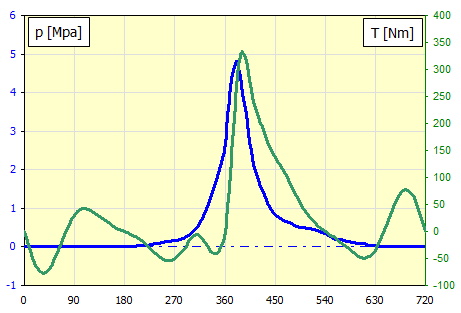### 1) Crank Mechanism

Define parameters of the crank mechanism in paragraph .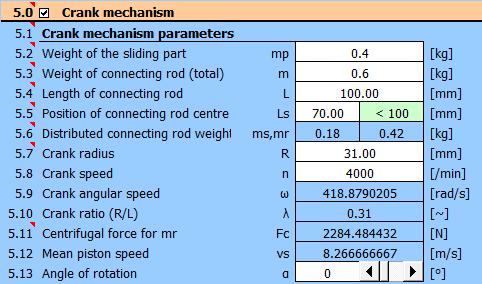From pressure and boring, we obtain the force Fp for each angle of rotation of the connecting rod.

Enter the angle α [°] and the calculated force into the table.

 α p Fp T ID [°] [Mpa] [N] [Nm] 1 0 0 0 0 2 10 0 0 -34.90 3 20 0 0 -62.31 4 30 0 0 -76.73 5 40 0 0 -76.02 6 50 0 0 -61.71 7 60 0 0 -38.28 8 70 0 0 -11.65 9 80 0 0 12.55 10 90 0 0 30.32 11 100 0 0 39.96 12 110 0 0 41.96 13 120 0 0 38.28 14 130 0 0 31.39 15 140 0 0 23.51 16 150 0 0 16.10 17 160 0 0 9.80 18 170 0 0 4.59 19 180 0 0 0.00 20 190 0.01 40 -4.67 21 200 0.02 81 -10.16 22 210 0.035 142 -17.12 23 220 0.05 203 -25.63 24 230 0.07 285 -35.46 25 240 0.09 366 -45.06 26 250 0.11 447 -52.22 27 260 0.13 529 -54.37 28 270 0.17 692 -51.77 29 280 0.2 814 -40.08 30 290 0.27 1099 -27.16 31 300 0.35 1425 -11.84 32 310 0.5 2035 -5.88 33 320 0.75 3053 -13.71 34 330 1.1 4478 -29.94 35 340 1.5 6107 -40.16 36 350 2 8143 -35.70 37 360 2.7 10993 0.00 38 370 4.1 16693 109.82 39 380 4.8 19543 265.61 40 390 4.2 17100 330.63 41 400 3.2 13028 306.88 42 410 2.3 9364 249.28 43 420 1.75 7125 212.30 44 430 1.4 5700 189.61 45 440 1.05 4275 157.11 46 450 0.85 3460 137.58 47 460 0.75 3053 123.13 48 470 0.66 2687 103.64 49 480 0.6 2442 83.51 50 490 0.54 2198 62.78 51 500 0.5 2035 44.80 52 510 0.47 1913 29.83 53 520 0.45 1832 17.91 54 530 0.4 1628 8.00 55 540 0.35 1425 0.00 56 550 0.28 1140 -6.98 57 560 0.2 814 -13.41 58 570 0.18 732 -21.35 59 580 0.15 610 -29.89 60 590 0.12 488 -38.36 61 600 0.1 407 -45.82 62 610 0.08 325 -49.42 63 620 0.05 203 -45.49 64 630 0.04 162 -35.34 65 640 0.02 81 -15.29 66 650 0.01 40 10.23 67 660 0 0 38.28 68 670 0 0 61.71 69 680 0 0 76.02 70 690 0 0 76.73 71 700 0 0 62.31 72 710 0 0 34.90 73 720 0 0 0.00

This gives us the torque "T" for each angle.

### 2) Flywheel

Required flywheel speed: 4000 /min
Required coefficient of fluctuation of speed: 0.01

Enter the angle and moment values in the table.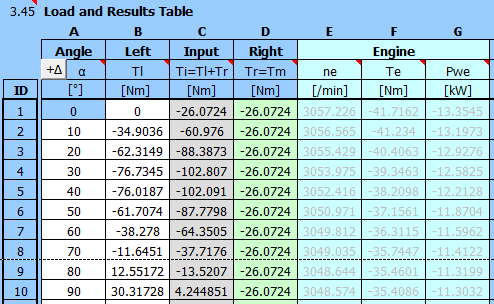Enter the required coefficient of fluctuation of speed and the required flywheel speed [3.3, 3.4].

Press the "Run A" button to get calculated the necessary moment of inertia of the flywheel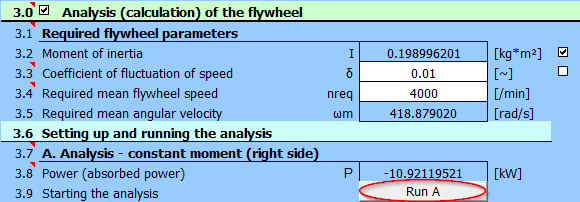The mean moment Tm is calculated. The moment is used as a constant load.

The results are shown immediately.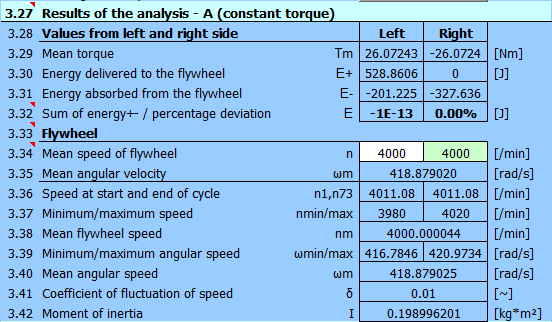and wide chart options.Here, for example, a graph of the motor torque and flywheel speed fluctuations.

## Example 2 - Design of a Flywheel for a Compressor Driven by an Induction Motor

The compressor is directly connected to the electric motor.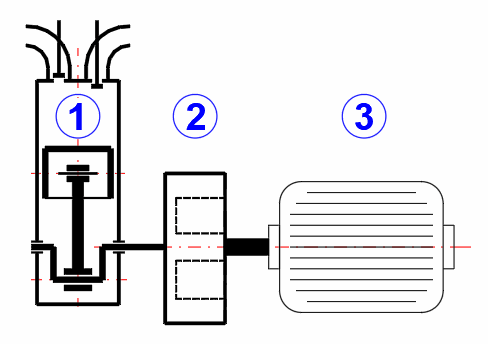Compressor parameters

Piston diameter: 30 mm
Connecting rod length: 60 mm
Connecting rod center of gravity: 40 mm
Piston weight: 0.0286 kg
Connecting rod weight: 0.0572 kg
Speed: 3000 /min
Pressure curve in the compressor

Using the calculation in chapter [5.0], we obtain a graph and a table of moments from the course of the pressure and the parameters of the crank mechanism.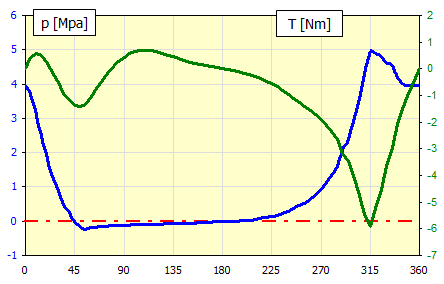Table of moments.

 α p Fp T ID [°] [MPa] [N] [Nm] 1 0 3.95 279.20905 0 2 5 3.73184519 263.78859 0.41128229 3 10 3.080272 217.7316 0.56473025 4 15 2.48848056 175.90033 0.51340057 5 20 1.8080703 127.80496 0.19717123 6 25 1.31442485 92.911218 -0.1398181 7 30 0.88581553 62.61461 -0.5128466 8 35 0.48195088 34.067101 -0.9203648 9 40 0.24031266 16.986701 -1.1441075 10 45 -0.00386229 -0.2730089 -1.3610845 11 50 -0.15132193 -10.696317 -1.4133192 12 55 -0.2362869 -16.702137 -1.3370272 13 60 -0.1973125 -13.947199 -1.0312084 14 65 -0.17359486 -12.270698 -0.7309989 15 70 -0.15544822 -10.987987 -0.4378542 16 75 -0.14173079 -10.018359 -0.1646365 17 80 -0.13402177 -9.4734403 0.0743766 18 85 -0.12725568 -8.9951742 0.27859924 19 90 -0.120625 -8.5264788 0.44357914 20 95 -0.11418232 -8.0710727 0.56667182 21 100 -0.10797454 -7.6322704 0.64817605 22 105 -0.10204254 -7.2129621 0.6908825 23 110 -0.09642109 -6.8156052 0.69948517 24 115 -0.09113888 -6.442228 0.67992578 25 120 -0.08621875 -6.0944443 0.63873909 26 125 -0.08167802 -5.7734789 0.58245836 27 130 -0.07629347 -5.3928677 0.51789065 28 135 -0.07066924 -4.995314 0.44958032 29 140 -0.06565042 -4.6405549 0.38115025 30 145 -0.06123852 -4.3286959 0.31567779 31 150 -0.05743152 -4.0595947 0.255123 32 155 -0.054225 -3.8329391 0.20044217 33 160 -0.05161318 -3.6483207 0.15175295 34 165 -0.04589893 -3.2444041 0.10900047 35 170 -0.031494 -2.2261796 0.07113676 36 175 -0.02287093 -1.6166511 0.03507503 37 180 -0.02 -1.4137167 4.9004E-17 38 185 -0.01808604 -1.2784271 -0.035273 39 190 -0.01233733 -0.8720747 -0.072752 40 195 -0.00273405 -0.1932585 -0.1146239 41 200 0.01075453 0.7601932 -0.1630174 42 205 0.02816664 1.9909827 -0.2199255 43 210 0.04954345 3.5020199 -0.2870821 44 215 0.07492346 5.2960275 -0.3657929 45 220 0.10433615 7.3750878 -0.4567331 46 225 0.13779491 9.7401485 -0.5597348 47 230 0.17528981 12.390507 -0.6735931 48 235 0.22517026 15.916348 -0.8019252 49 240 0.29328125 20.73083 -0.9484907 50 245 0.36708323 25.947585 -1.101615 51 250 0.44631634 31.548243 -1.256091 52 255 0.53063809 37.508596 -1.4057648 53 260 0.61961808 43.798221 -1.5438906 54 265 0.75455803 53.336564 -1.7190622 55 270 0.91875 64.942611 -1.9129609 56 275 1.11767048 79.003471 -2.1337469 57 280 1.34076178 94.772866 -2.3653236 58 285 1.60625075 113.53918 -2.6341702 59 290 2.0520672 145.05208 -3.1634106 60 295 2.38544142 168.61692 -3.4715845 61 300 2.88710938 204.07774 -4.0038618 62 305 3.57144831 252.4508 -4.7586566 63 310 4.29339033 303.48188 -5.4628709 64 315 4.94613771 349.62187 -5.9198089 65 320 4.88350703 345.19477 -5.2300665 66 325 4.81539388 340.38014 -4.512448 67 330 4.62145388 326.67133 -3.6522527 68 335 4.53092919 320.27251 -2.9430483 69 340 4.19706534 296.67307 -2.0759393 70 345 3.98461327 281.65572 -1.4133487 71 350 3.95 279.20905 -0.918416 72 355 3.95 279.20905 -0.4560135 73 360 3.95 279.20905 -1.277E-15

First, fill in the angles α and the corresponding moments in the table [3.43].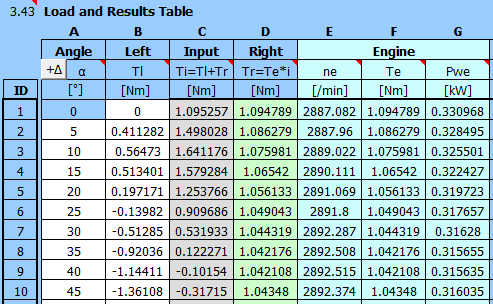Enter the flywheel parameters:
Coefficient of fluctuation of speed: 0.01
Flywheel speed: 3000 /min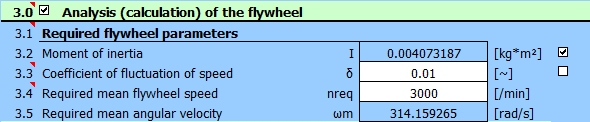And the motor parameters:
Two-pole motor, ns: 3000 /min
Power from the help table: P=0.55kW
Set the gear ratio to: i=1.0

Run analysis B (several times in a row).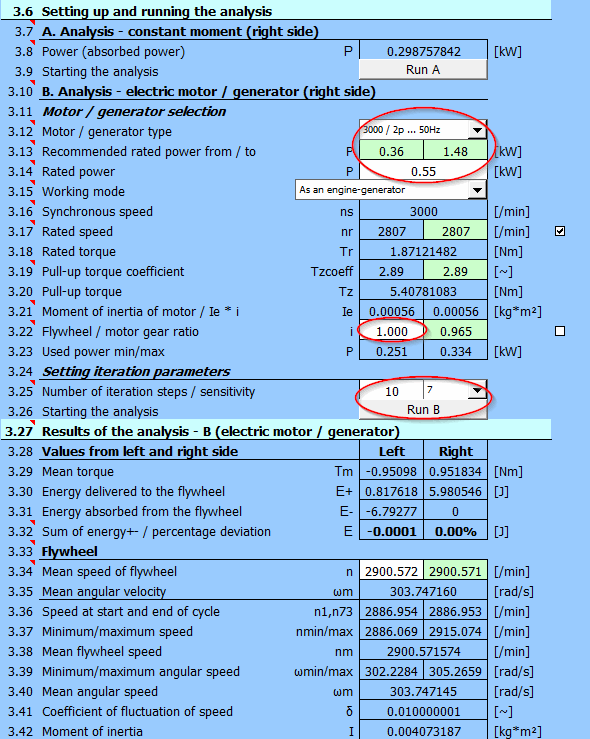With a direct motor-compressor connection, it is not possible to achieve a synchronous speed of 3000 /min, because the asynchronous motor has zero power at synchronous speed. Due to the load, the speed thus stabilizes at a medium speed of 2900 rpm.

Graph of the motor performance and speed oscillation near the middle speed (2900 /min) during the work cycle: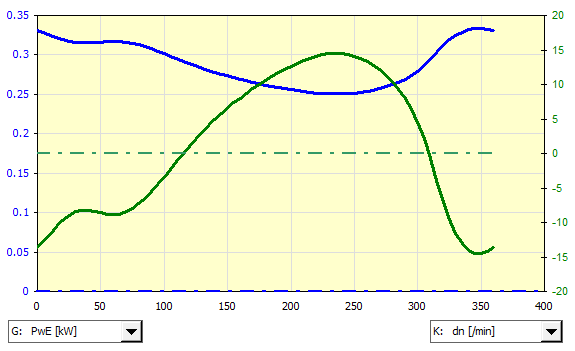## Example 3 - Design of a Flywheel for a Crank Press Driven by an Induction Motor

The crank mechanism (1) is connected via a 5:1 gear (2) to the flywheel (3). The flywheel is driven via a belt transmission (4) by an asynchronous electric motor (5).

- Determine the moment of inertia of the flywheel for the specified run unevenness
- Determine the required power of the electric motor
- Propose the dimensions of the flywheel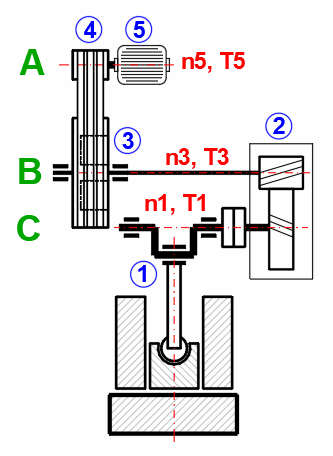### 1) Crank Press (Mechanism):

Required force: 10 tons
Working path: 20mm
Strokes per minute: 30
Weight of sliding part: 30 kg
Weight of the connecting rod: 30 kg
Connecting rod length 400mm
Connecting rod center of gravity: 200mm
Resistance forces: 200NIn paragraph [5.0], define the parameters of the crank mechanism.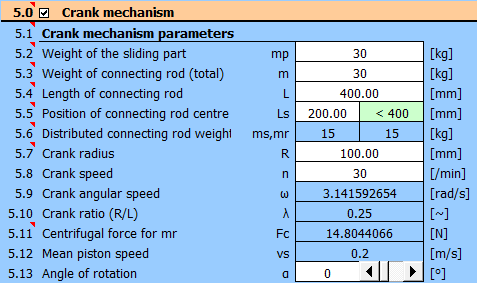In the table, set the step to 5 degrees and enter the load values.
Against the loading force of 10 tons ~ 100000N, the forces of weight and resistance (30kg+30kg+-200N)=800N (400N) act throughout the entire revolution.
100000N force acts from 325 degrees (22mm from top dead center).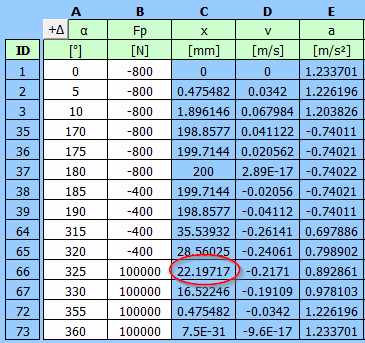A detailed table with the entered data of the torque on the crank T1 and the torque in front of the 5:1 gearbox T3.

 α Fp T1 T3=T1/5 ID [°] [N] [Nm] [Nm] 1 0 -800 0 0 2 5 -800 -11.16588081 -2.233176162 3 10 -800 -22.13614645 -4.427229291 4 15 -800 -32.72117313 -6.544234626 5 20 -800 -42.74303421 -8.548606841 6 25 -800 -52.04065447 -10.40813089 7 30 -800 -60.4741842 -12.09483684 8 35 -800 -67.92841631 -13.58568326 9 40 -800 -74.31513183 -14.86302637 10 45 -800 -79.57432571 -15.91486514 11 50 -800 -83.67433049 -16.7348661 12 55 -800 -86.61091605 -17.32218321 13 60 -800 -88.40549241 -17.68109848 14 65 -800 -89.1025794 -17.82051588 15 70 -800 -88.76672722 -17.75334544 16 75 -800 -87.47907757 -17.49581551 17 80 -800 -85.33374615 -17.06674923 18 85 -800 -82.43418681 -16.48683736 19 90 -800 -78.8896695 -15.7779339 20 95 -800 -74.81197108 -14.96239422 21 100 -800 -70.3123455 -14.0624691 22 105 -800 -65.49881019 -13.09976204 23 110 -800 -60.47376161 -12.09475232 24 115 -800 -55.33191717 -11.06638343 25 120 -800 -50.1585722 -10.03171444 26 125 -800 -45.0281604 -9.005632079 27 130 -800 -40.0031109 -8.00062218 28 135 -800 -35.13300373 -7.026600745 29 140 -800 -30.45403455 -6.090806909 30 145 -800 -25.98880731 -5.197761462 31 150 -800 -21.74647679 -4.349295357 32 155 -800 -17.72326121 -3.544652241 33 160 -800 -13.90333756 -2.780667512 34 165 -800 -10.26011853 -2.052023707 35 170 -800 -6.757892469 -1.351578494 36 175 -800 -3.353787386 -0.670757477 37 180 -800 -4.69655E-15 -9.39309E-16 38 185 -400 1.604039453 0.320807891 39 190 -400 3.232166795 0.646433359 40 195 -400 4.90735673 0.981471346 41 200 -400 6.650407922 1.330081584 42 205 -400 8.478975168 1.695795034 43 210 -400 10.40673082 2.081346165 44 215 -400 12.44267607 2.488535213 45 220 -400 14.59060769 2.918121538 46 225 -400 16.84873248 3.369746496 47 230 -400 19.20941071 3.841882141 48 235 -400 21.65900483 4.331800966 49 240 -400 24.17781008 4.835562017 50 245 -400 26.74005012 5.348010024 51 250 -400 29.31393288 5.862786575 52 255 -400 31.86177714 6.372355427 53 260 -400 34.34023682 6.868047363 54 265 -400 36.70066494 7.340132987 55 270 -400 38.8896695 7.777933901 56 275 -400 40.84991711 8.169983422 57 280 -400 42.52123459 8.504246919 58 285 -400 43.84204452 8.768408904 59 290 -400 44.75114629 8.950229258 60 295 -400 45.18982349 9.037964697 61 300 -400 45.10422222 9.020844444 62 305 -400 44.44790807 8.889581614 63 310 -400 43.18447524 8.636895048 64 315 -400 41.29005446 8.258010892 65 320 -400 38.75554992 7.751109983 66 325 100000 -8081.747466 -1616.349493 67 330 100000 -7161.909833 -1432.381967 68 335 100000 -6138.383421 -1227.676684 69 340 100000 -5024.644787 -1004.928957 70 345 100000 -3836.174802 -767.2349603 71 350 100000 -2590.128246 -518.0256491 72 355 100000 -1304.957414 -260.9914828 73 360 100000 -3.6734E-12 -7.34681E-13

### 2) Gearbox

Gear ratio is 5:1. Torque values can be found in the previous table. Flywheel revolutions = revolutions at the input to the gearbox n3 = 30 * 5 = 150 /min.

### 3) Flywheel

Required coefficient of fluctuation of speed: 0.03
Required flywheel revolutions 150 /minEnter the required coefficient of fluctuation of speed and the required flywheel speed [3.3, 3.4].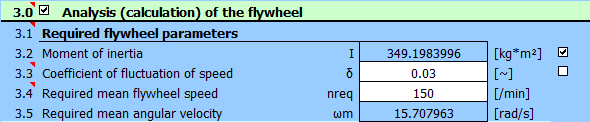#### Completing the Table of Moments

Since the ratio between the crank mechanism and the flywheel is 5:1, the flywheel turns 5 times for one revolution of the crank mechanism (1 working cycle). The division of the angle in the calculation of the crank mechanism was 5°, the division in the flywheel analysis table will thus be 5 * 5 = 25°

It is then possible to directly copy the T3 torque values into the second column (B).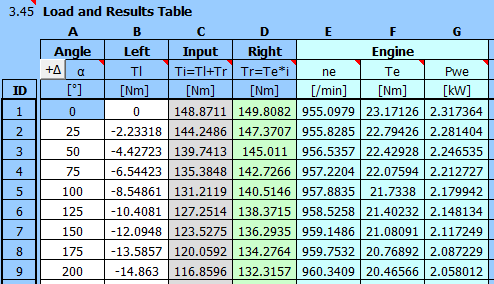#### Input Data and Analysis

Under Motor/Generator selection [3.12], select the motor 1000 /min. The recommended rated power [3.13] is estimated based on the torque curve (column B).

Choose from the range of 3kW motors [3.14].

The flywheel: motor gear ratio (belt drive) is set automatically [3.22] so that the required flywheel revolutions are achieved.

Set the number of approximation steps to 10 and the sensitivity to 5 [3.25].

Press the "Start B" button. 10 steps will take place in sequence, during which the motor torque is repeatedly calculated from the flywheel revolutions. The value of the sum of the energies of the right and left sides [3.32] should decrease (reach the equilibrium state). You can press the button several times in a row to refine the results.

At the same time, the relevant moment of inertia is calculated [3.42].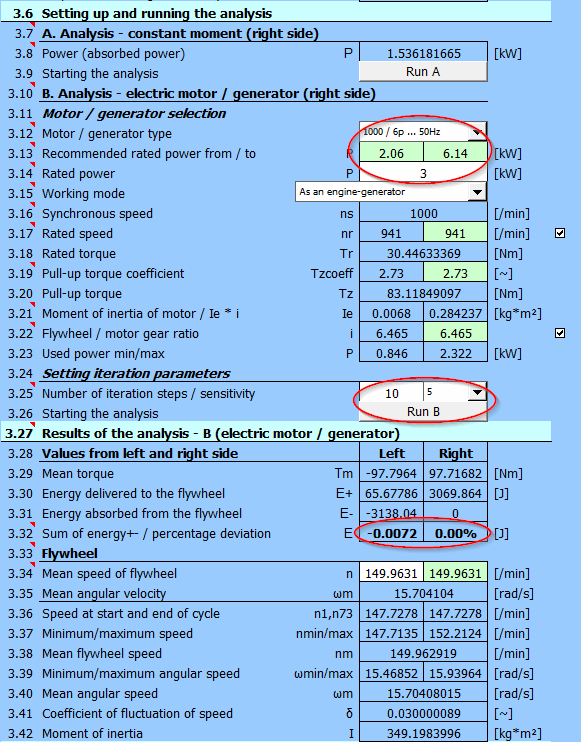### 4) Design of Flywheel Dimensions

In paragraph [2.0], enter the moment of inertia from the previous step, the density, the b/h ratio, and the inner diameter.

###### Note: If necessary, it is possible to include all rotating masses in the calculation and recalculate their moments of inertia using table [2.25].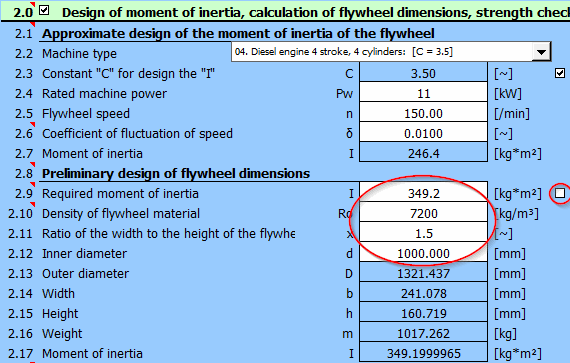The flywheel ring parameters are on lines [2.13-2.17]

## Setting calculations, change the language.

Information on setting of calculation parameters and setting of the language can be found in the document "Setting calculations, change the language".

## Workbook (calculation) modifications.

General information on how to modify and extend calculation workbooks is mentioned in the document "Workbook (calculation) modifications".

## List of Standards, literature list:

Literature:
 Strojně technická příručka (Svatopluk Černoch)
 Shigley’s Mechanical Engineering Design
 Strojírenská příručka (1992)

Machinery’s Handbook (26th Edition)

Standards:
ČSN EN 60034-30-1 :20 14
EN 60034-30-1 :20 14
Rotating electrical machines — Part 30-1: Efficiency classes of line operated AC motors (IE code)
Machines Olectriques tournantes — Partie 30-1: Classes de rendement pour les moteurs a courant alternatif alimentés par le réseau (Code IE)
Drehende elektrische Maschinen — Teil 30-1: Wirkungrad-Klassifizierung von netzgespeisten Drehstrommotoren (IE-Code)

Catalogues and company materials:
Siemens
ABB Automation Products

^